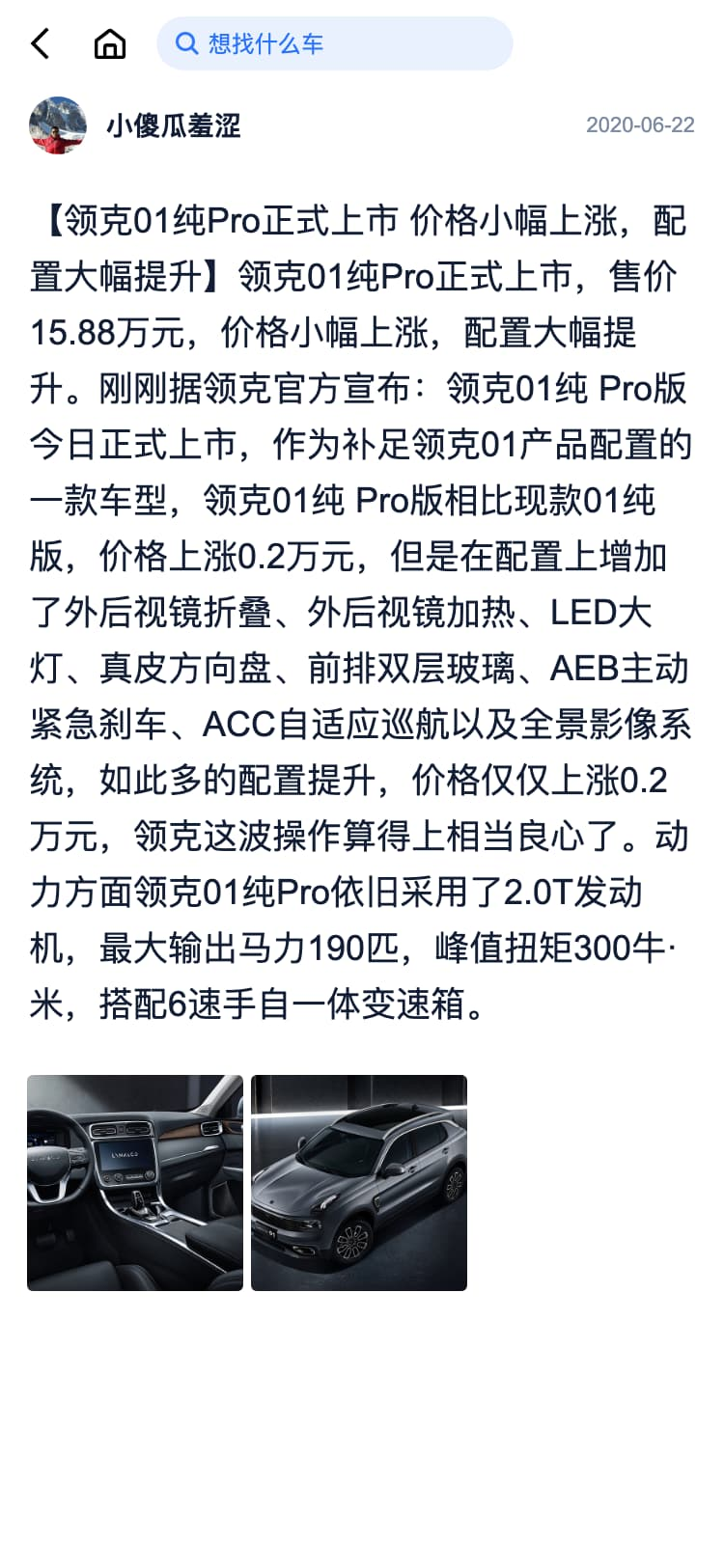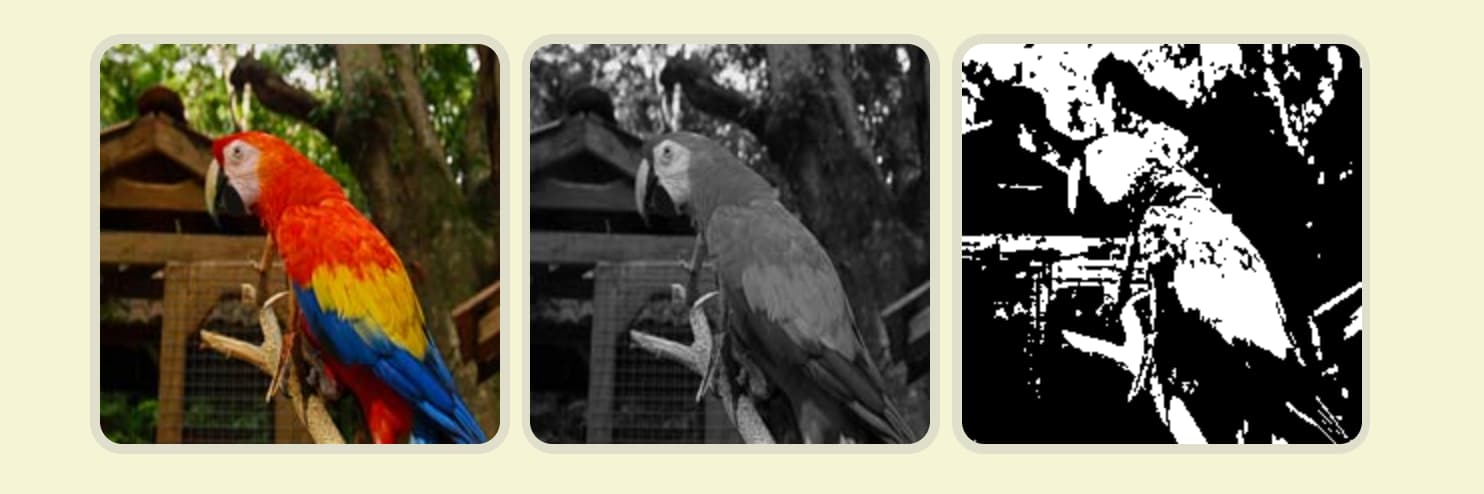# 如何利用图片对比算法处理白屏检测

### 常见的图片相似度方案比较

1. hash 算法

将每个图片生成一个哈希值，最终比较两张图片的哈希值，结果越接近，图片越相似。

• aHash：平均值哈希。速度比较快，但是常常不太精确。

• pHash：感知哈希。精确度比较高，但是速度方面较差一些。

• dHash：差异值哈希。精确度较高，且速度也非常快。

2.ssim(structural similarity)算法

SSIM（structural similarity）结构相似度算法，一种全参考的图像质量评价指标，分别通过对图片亮度、对比度、结构进行对比，一般是用来评估图片压缩后的质量

3.PSNR: （Peak Signal to Noise Ratio）峰值信噪比算法

4.histogram 算法

• Correlation 相关性比较
• Chi-Square 卡方比较
• Intersection 十字交叉性
• Bhattacharyya distance 巴氏距离

### 实践temp1.png: 几乎完全白屏

temp2.png: 中间主要内容白屏

temp3.png: 内容丰富，完全不白屏

temp4.png: 内容一般，不白屏

1. ##### ssim 算法

ssim 使用的是`compare_ssim`这个库。

``````from SSIM_PIL import compare_ssim
from PIL import Image

def compare_image_in_ssim(imgpath1, imgpath2):
image1 = Image.open(imgpath1)
image2 = Image.open(imgpath2)
value = compare_ssim(image1, image2)
return value
``````

1. ##### histogram 算法

``````import cv2 as cv

def histogram(src_base, src_test1):
## [Convert to HSV]
hsv_base = cv.cvtColor(src_base, cv.COLOR_BGR2HSV)
hsv_test1 = cv.cvtColor(src_test1, cv.COLOR_BGR2HSV)
## [Using 50 bins for hue and 60 for saturation]
h_bins = 50
s_bins = 60
histSize = [h_bins, s_bins]

# hue varies from 0 to 179, saturation from 0 to 255
h_ranges = [0, 180]
s_ranges = [0, 256]
ranges = h_ranges + s_ranges # concat lists

# Use the 0-th and 1-st channels
channels = [0, 1]
## [Using 50 bins for hue and 60 for saturation]

## [Calculate the histograms for the HSV images]
hist_base = cv.calcHist([hsv_base], channels, None, histSize, ranges, accumulate=False)
cv.normalize(hist_base, hist_base, alpha=0, beta=1, norm_type=cv.NORM_MINMAX)

hist_test1 = cv.calcHist([hsv_test1], channels, None, histSize, ranges, accumulate=False)
cv.normalize(hist_test1, hist_test1, alpha=0, beta=1, norm_type=cv.NORM_MINMAX)

## [Calculate the histograms for the HSV images]

res = []
## [Apply the histogram comparison methods]
for compare_method in range(4):
base_base = cv.compareHist(hist_base, hist_base, compare_method)
base_test1 = cv.compareHist(hist_base, hist_test1, compare_method)
data = {"method": compare_method, "base_base": base_base, "base_test1": base_test1}
res.append(data)
## [Apply the histogram comparison methods]
``````

``````{
"Correlation": {
"temp1.png": 0.9986657002039772,
"temp2.png": 0.9999985026552713,
"temp3.png": 0.9975293986570175,
"temp4.png": 0.9999895931732444
},
"Chi-Square": {
"temp1.png": 0,
"temp2.png": 0,
"temp3.png": 0,
"temp4.png": 0
},
"Intersection": {
"temp1.png": 1,
"temp2.png": 1,
"temp3.png": 1,
"temp4.png": 1
},
"Bhattacharyya distance": {
"temp1.png": 0.1818559920237636,
"temp2.png": 0.04000132137383061,
"temp3.png": 0.3436786429149872,
"temp4.png": 0.12853959709846752
}
}
``````

• Correlation 相关性比较 ： 结果越接近 1 越相似；
• Chi-Square 卡方比较： 结果越接近 0 越相似；
• Intersection 十字交叉性： 结果越接近 1 越相似；
• Bhattacharyya distance 巴氏距离： 结果越接近 0 越相似；ssim 算法主要是用来评估图片质量，反映图片失真程度的，用在白屏检测这里，用途也不是很“正当”。剩下 histogram 算法，主要是先生成颜色的直方图，再进行比较来代替图片本身的比较。一般来说正常的网页肯定是图文并茂的，直方图应该也比较丰富；白屏的网页，直方图的结果应该是比较单一的。

#### 最终解决方案``````import cv2 as cv
def otsu_histogram(img, img1):
dst = cv.cvtColor(img, cv.COLOR_BGR2GRAY)
dst1 = cv.cvtColor(img1, cv.COLOR_BGR2GRAY)
ret,th = cv.threshold(dst1,0,255,cv.THRESH_BINARY+cv.THRESH_OTSU)

hist_base = cv.calcHist([dst], , None, , (0, 256), accumulate=False)
hist_test1 = cv.calcHist([th], , None, , (0, 256), accumulate=False)

cv.normalize(hist_base, hist_base, alpha=0, beta=1, norm_type=cv.NORM_MINMAX)
cv.normalize(hist_test1, hist_test1, alpha=0, beta=1, norm_type=cv.NORM_MINMAX)

res = []
## [Apply the histogram comparison methods]
for compare_method in range(4):
base_base = cv.compareHist(hist_base, hist_base, compare_method)
base_test1 = cv.compareHist(hist_base, hist_test1, compare_method)
data = {"method": compare_method, "base_base": base_base, "base_test1": base_test1}
res.append(data)
return res
``````

``````{
"Correlation": {
"temp1.png": 0.999998199473158,
"temp2.png": 0.9999303693978338,
"temp3.png": 0.9807698254660822,
"temp4.png": 0.9994585418568566
},
"Chi-Square": {
"temp1.png": 0.0,
"temp2.png": 0.0,
"temp3.png": 0.0,
"temp4.png": 0.0
},
"Intersection": {
"temp1.png": 1.0,
"temp2.png": 1.0,
"temp3.png": 1.0,
"temp4.png": 1.0
},
"Bhattacharyya distance": {
"temp1.png": 0.03078108788995894,
"temp2.png": 0.07647753684216813,
"temp3.png": 0.2944281499295871,
"temp4.png": 0.12674005983573874
}
}
``````

``````def is_white_page(img, img1, threadhold=0.08):
'''
大律法+直方图： 判断是否白屏
Args:
img: 白色的图片
img1: 对比的图片
Return: Boolean ，跟白屏的相似度小于阈值返回true
'''
dst = cv.cvtColor(img, cv.COLOR_BGR2GRAY)
dst1 = cv.cvtColor(img1, cv.COLOR_BGR2GRAY)
ret,th = cv.threshold(dst1,0,255,cv.THRESH_BINARY+cv.THRESH_OTSU)

hist_base = cv.calcHist([dst], , None, , (0, 256), accumulate=False)
hist_test1 = cv.calcHist([th], , None, , (0, 256), accumulate=False)

cv.normalize(hist_base, hist_base, alpha=0, beta=1, norm_type=cv.NORM_MINMAX)
cv.normalize(hist_test1, hist_test1, alpha=0, beta=1, norm_type=cv.NORM_MINMAX)

base_test1 = cv.compareHist(hist_base, hist_test1, 3)
return True
else:
return False
``````

（一）网页 header 跟 footer 内容比较丰富 ，但是 body 为空的

（二）网页背景色较深的``````def is_white_page(img, img1, threadhold=0.08):
'''
大律法+直方图： 判断是否白屏
Args:
img: 白色的图片
img1: 对比的图片
Return: Boolean ，跟白屏的相似度小于阈值返回true
'''
img1 = clip(img1)
img = clip(img)
dst = cv.cvtColor(img, cv.COLOR_BGR2GRAY)
dst1 = cv.cvtColor(img1, cv.COLOR_BGR2GRAY)
ret,th = cv.threshold(dst1,0,255,cv.THRESH_BINARY+cv.THRESH_OTSU)

hist_base = cv.calcHist([dst], , None, , (0, 256), accumulate=False)
hist_test1 = cv.calcHist([th], , None, , (0, 256), accumulate=False)

cv.normalize(hist_base, hist_base, alpha=0, beta=1, norm_type=cv.NORM_MINMAX)
cv.normalize(hist_test1, hist_test1, alpha=0, beta=1, norm_type=cv.NORM_MINMAX)

base_test1 = cv.compareHist(hist_base, hist_test1, 3)
return True
else:
return False

def clip(img, size ={'top': 160, 'bottom': 140}):
'''
裁剪图片
top: 裁掉顶部区域的大小
bottom:裁掉顶部区域的大小
'''
sz1 = img.shape         #图像的高度（行 范围）
sz2 = img.shape         #图像的宽度（列 范围）

a=size['top'] # y start
b=sz1-size['bottom'] # y end
c=0 # x start
d=sz2 # x end
return img[a:b,c:d]  #裁剪后的图像
``````